Author: Oscar Cronquist Article last updated on May 03, 2019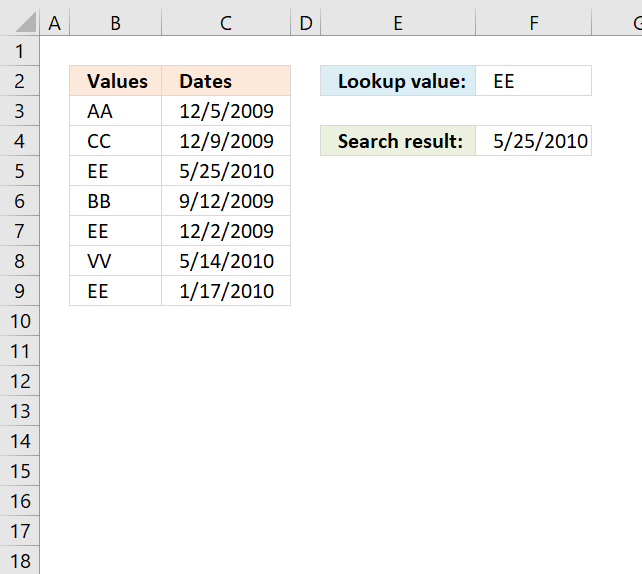### Lookup a value and find max date

The picture below shows you values in column B (B3:B9) and dates in column C (C3:C9). The formula in cell F4 lets you search for value and return the latest date in an adjacent or corresponding column for that value.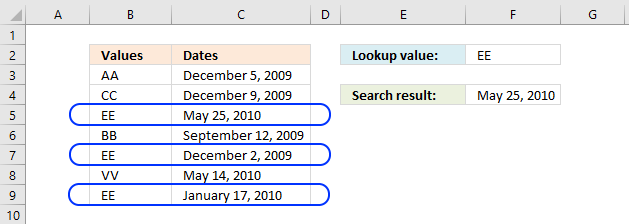Update, 2017-08-15! Added a regular formula.

Formula in cell F4:

=MAX(INDEX((C3=A8:A14)*B8:B14,))

Array formula in F4:

=MAX(IF(C3=A8:A14, B8:B14))

Formula in cell F4 (Excel 2016):

=MAXIFS(C3:C9,B3:B9,F2)

#### Watch a video where I explain the formulas

Recommended article:

Lookup the nearest date

The image above demonstrates an array formula in cell E4 that searches for the closest date in column A to the […]

#### How to create an array formula

1. Double click cell C5
2. Copy / Paste above array formula
3. Press and hold Ctrl + Shift simultaneously
4. Press Enter
5. Release all keys

The formula changes and now begins and ends with a curly bracket, don't enter these characters yourself. They appear automatically.

Recommended article:

How to enter an array formula

Array formulas allows you to do advanced calculations not possible with regular formulas.

#### Explaining array formula in cell C5

You can follow along if you select cell C5 and go to tab "Formulas" on the ribbon and then click "Evaluate Formula" button. Click "Evaluate" button, shown on the dialog box, to move to next step.

Step 1 - Find values equal to lookup value

C3=A8:A14

becomes

"EE"={"AA";"CC";"EE";"BB";"EE";"VV";"EE"}

and returns

{FALSE;FALSE;TRUE;FALSE;TRUE;FALSE;TRUE}

Step 2 - Convert boolean values to corresponding dates

IF(C3=A8:A14, B8:B14)

becomes

IF({FALSE;FALSE;TRUE;FALSE;TRUE;FALSE;TRUE} ,B8:B14)

becomes

IF({FALSE;FALSE;TRUE;FALSE;TRUE;FALSE;TRUE} , {40152; 40156; 40323; 40068; 40149; 40312; 40195})

and returns

{FALSE;FALSE;40323;FALSE;40149;FALSE;40195}

Step 3 - Return the largest value

=MAX(IF(C3=A8:A14, B8:B14))

becomes

=MAX({FALSE;FALSE;40323;FALSE;40149;FALSE;40195})

and returns 40323 formatted as 2010-05-25.

* You will also get a weekly newsletter, unsubscribe anytime!

### Lookup a value and find max date (Pivot Table)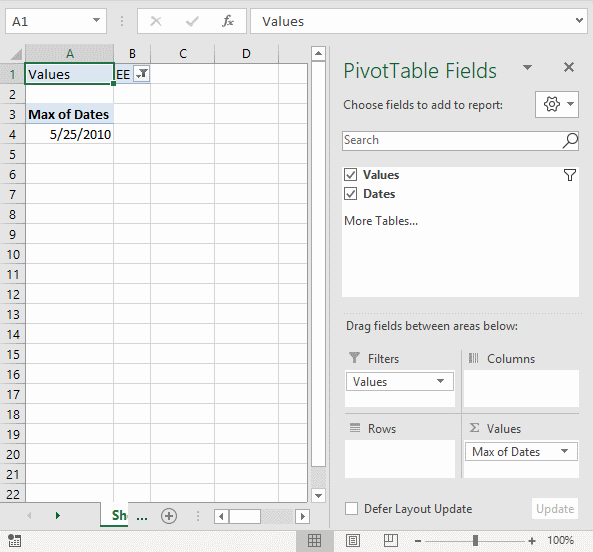The formulas demonstrated in this article may be too slow or taking too much memory if you work with huge amounts of data. The Pivot Table is an excellent option in such cases, it is remarkably fast even with lots of data.

#### How to set up Pivot Table

1. Select the cell range containing the data.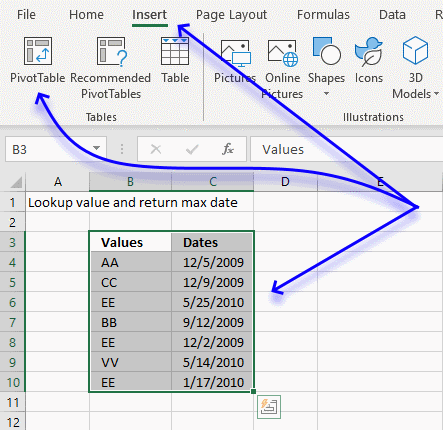2. Go to tab "Insert" on the ribbon.
3. Click on "Pivot Table" button.
4. A dialog box appears.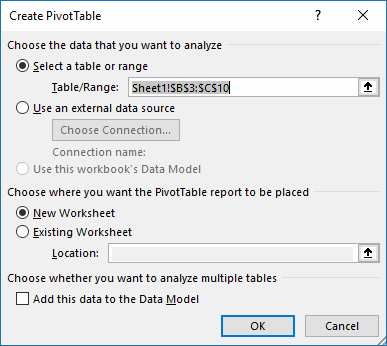I usually place the Pivot Table on a new worksheet so it doesn't hide parts of the data set while filtering etc.
5. Click OK button.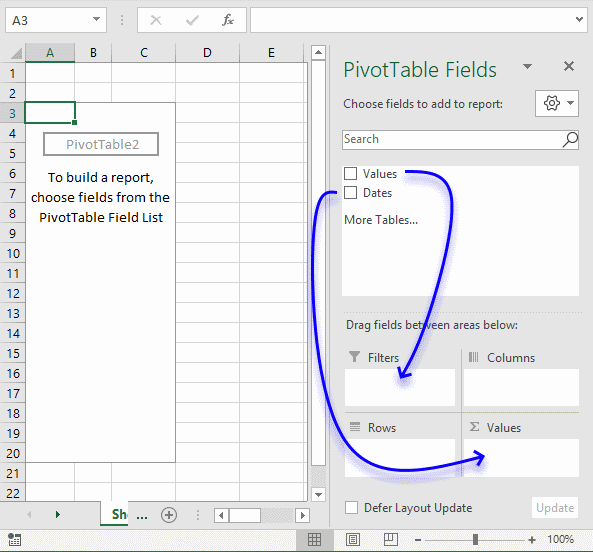1. Click on Values and drag to Filters field, see blue arrow above.
2. Click on Dates and drag to Values field.
3. Click on "Count of Dates".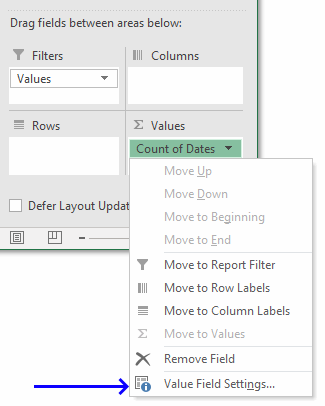4. Click on "Value Field Settings...".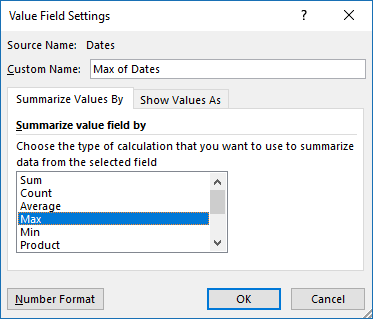5. Click on "Max" to select it.
6. Click on "Number Format" button.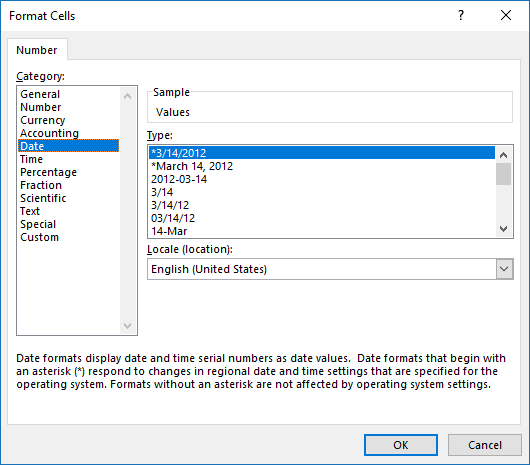7. Click on category "Date" and select a type.
8. Click OK button.
9. Click OK button.Click on cell B1 to filter the latest date based on the selected value, the image above shows value EE selected and the latest date based on that value is 5/25/2010.

* You will also get a weekly newsletter, unsubscribe anytime!

### Lookup all values and find latest (earliest) date

The following formula looks in column C for the most recent date for each value in column B.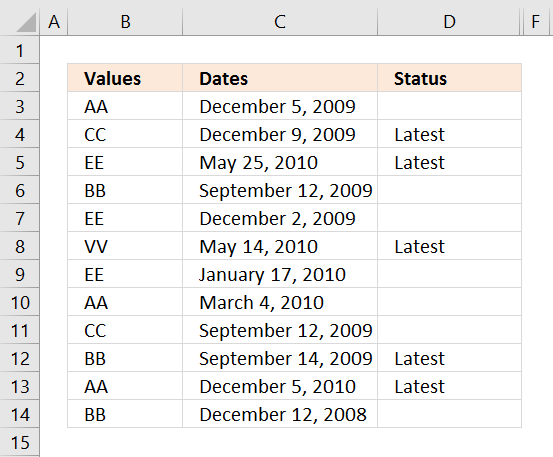Formula in cell D3:

=IF(MAX(INDEX((B3=\$B\$3:\$B\$14)*\$C\$3:\$C\$14,))=C3,"Latest","")

Array formula in cell D3:

=IF(MAX(IF(B3=\$B\$3:\$B\$14, \$C\$3:\$C\$14))=C3, "Latest", "")

How to create an array formula

Formula in cell D3:

=IF(MAXIFS(\$C\$3:\$C\$14,\$B\$3:\$B\$14,B3)=C3,"Latest","")

#### Watch a video explaining the formula above

How to copy array formula

1. Copy cell C2
2. Select cell range C3:C8
3. Paste

* You will also get a weekly newsletter, unsubscribe anytime!

### Lookup and find last date using multiple conditionsFormula in cell H3:

=MAX(INDEX((C2:C29=H1)*(D2:D29=H2)*E2:E29,))

Array formula in cell H3:

=MAX(IF((C2:C29=H1)*(D2:D29=H2),E2:E29,""))

How to create an array formula

### Lookup and find latest date on multiple sheets

The following picture shows you a workbook with 4 worksheets. The formula in cell B3 looks for the latest date in all three worksheets using the condition in cell B2.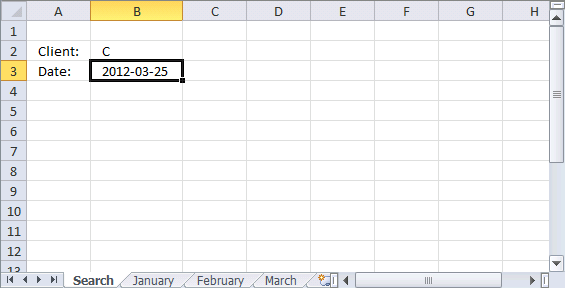Array formula in cell B3:

=MAX(IF(B2=January!\$B\$2:\$B\$10, January!\$A\$2:\$A\$10, ""), IF(B2=February!\$B\$2:\$B\$10, February!\$A\$2:\$A\$10, ""), IF(B2=March!\$B\$2:\$B\$10, March!\$A\$2:\$A\$10, ""))

* You will also get a weekly newsletter, unsubscribe anytime!

### Lookup and find latest date, return corresponding value on same row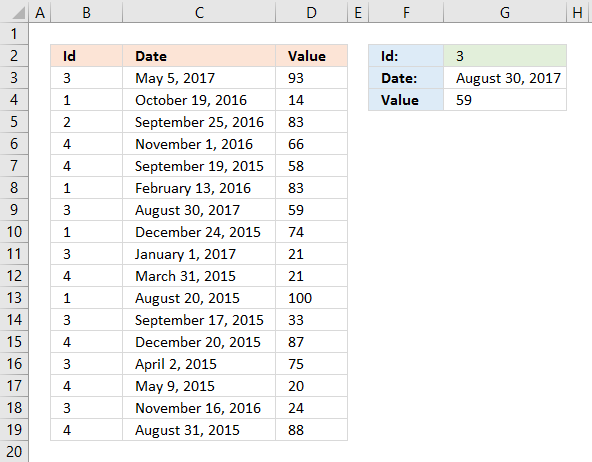Enter a quarter in cell G3.

Array formula in cell G3:

=MAX((B3:B19=G2)*C3:C19)

If you prefer a regular formula in G3:

=MAX(INDEX((B3:B19=G2)*C3:C19,))

Formula in cell G4:

=INDEX(\$D\$3:\$D\$19, SUMPRODUCT((B3:B19=G2)*(G3=C3:C19)*MATCH(ROW(B3:B19), ROW(B3:B19))))

#### Watch a video explaining the formulas above

IF(logical_test, [value_if_true], [value_if_false])
Checks whether a condition is met, and returns one value if TRUE, and another value if FALSE

INDEX(array,row_num,[column_num])
Returns a value or reference of the cell at the intersection of a particular row and column, in a given range

MATCH(lookup_value,lookup_array, [match_type])
Returns the relative position of an item in an array that matches a specified value

MAX(number1,[number2],..)
Returns the largest value in a set of values. Ignores logical values and text.